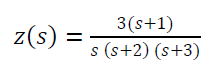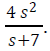41. Consider the following function:The final value of 𝑧 (𝑡 ) will be:

(a) 1/2

(b) 3/2

(c) 1/3

(d) 2/3

42. The current through a circuit element isThe current in 𝑡 domain at 𝑡 →∞ and 𝑡 →0 will be respectively:

(a) Zero and One

(b) Zero and Infinity

(c) Infinity and Zero

(d) Infinity and One

43. Which one of the following networks is called a ladder network?

(a) Non-recurrent network

(b) Distributed network

(c) Recurrent network

(d) Passive network

44. In hybrid parameter representation, the voltage of the input port and the current of the output port are expressed in terms of the:

(a) Voltage of input port and current of output port

(b) Current of input port and voltage of output port

(c) Voltage of input port and current of input port

(d) Current of output port and voltage of output port

45. A series circuit has 𝑅=4 Ω and 𝐿 =0.01 𝐻 . Its impedance at 100 Hz will be nearly:

(a) 7.45 ∠ 37.5° Ω

(b) 7.45 ∠ 57.5° Ω

(c) 8.25 ∠ 37.5° Ω

(d) 8.25 ∠ 57.5° Ω

46. In a series RLC circuit 𝑅=3 Ω, X𝐿 =𝑗 6Ω,
X=−𝑗 2Ω. If the current is 10 ∠−143° A, the voltage applied across the combination will be:

(a) 50 ∠−90° V

(b) 50 ∠90° V

(c) 25 ∠−90° V

(d) 25 ∠90° V

47. In a series 𝑅-𝐿 circuit, 𝑅=20 Ω, while 𝐿 = 60 𝑚H. The input current lags the supply voltage by 60° . The value of applied frequency will be nearly:

(a) 91.9 Hz

(b) 87.7 Hz

(c) 82.7 Hz

(d) 78.9 Hz

48. The charge carrier transit time is 70 ns for a silicon diode of 10 mA forward current. The diffusion capacitance will be:

(a) 1 nF

(b) 3 nF

(c) 5 nF

(d) 7 nF

49. A 6-bit DAC has a step size of 50 mV. The percentage resolution will be nearly:

(a) 2.8 %

(b) 2.4 %

(c) 1.6 %

(d) 1.2 %